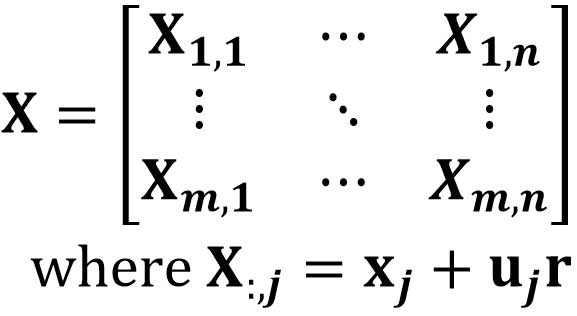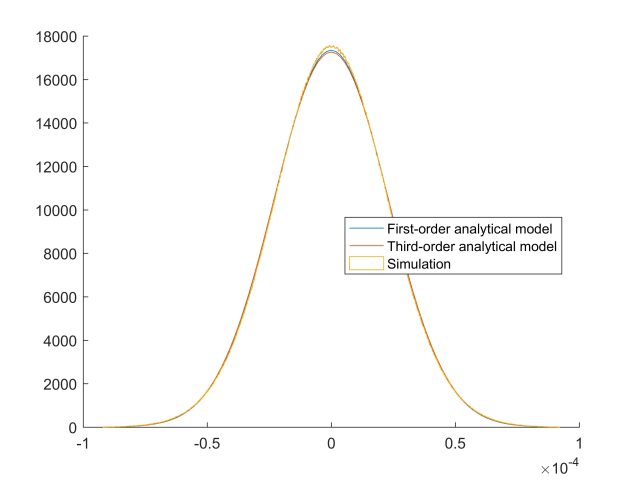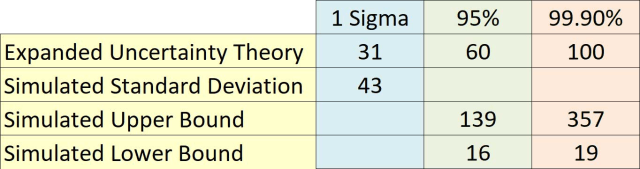Why Simulation Is the Future of Uncertainty Evaluation
Dr Jody Muelaner posted on November 12, 2018 |
Non-linearity issues with analytical methods for uncertainty of measurement.In this article I will show that the conventional method for calculating uncertainty is not always reliable. In fact, it is generally only exact when the measurement can be represented by a simple linear equation and the input uncertainties are all normally distributed. Whenever the measurement is more complex, there will be errors in the way uncertainties are combined. Using the conventional analytic methods, these errors can be difficult to quantify, although there are some methods which can be used. I will also show simulation can be a much more reliable approach.

Uncertainty evaluation requires a mathematical model describing how influences on the measurement combine to produce the measurement result. The uncertainty in each influence is then combined, with reference to the mathematical model, to give the uncertainty in the measurement result. This approach enables all available experimental data to be combined with other unobserved sources of uncertainty, which we know about by some other means. In a previous article I showed how gage studies can underestimate uncertainty by failing to include these Type-B uncertainties.

I will start with a simple example of a linear measurement model, showing that this gives an exact solution. I will then show what happens with more complex models and compare the results with simulations. With simulation, we can quantify the errors in the analytical approach.

## Uncertainty Evaluation for Linear Models

Let’s consider the simplest of measurement models with just two influences which add up to give the measurement result. These could be the repeatability of the instrument and its calibrated value. The measurement result is denoted y and the two influences a and b, so we can say that the measurement result is given by:

y = a + b

Since this is a linear combination, if a is increased by one unit, then y will increase by one unit. This is independent of the value of b. The same can be said for a change in b. In other words, the partial derivatives, or sensitivity coefficients, are all equal to one. Applying the law of propagation of uncertainty, the uncertainty of the measurement result is therefore simply given by:

u2(y) = u2(a) + u2(b)

If u(a) and u(b) are normally distributed, then u(y) will also be normally distributed and this will be an exact solution. If, however, u(a) and u(b) are uniformly distributed, and of equal magnitude, then u(y) will follow a triangular distribution. If the inputs are some other combination of distributions, then the output distribution cannot readily be determined without simulation.

## Simulating Uncertainty

I will now briefly explain how measurements are simulated and the results used to prove the accuracy of analytical solutions. I will explain the ins and outs of Monte Carlo simulation in a future article. In essence, simulation involves calculating our measurement function many times but in each case adding some randomly generated error to each influence quantity. We then have many simulated measurement results and can calculate the standard deviation of these, just as we would if they were actual experimental measurement results.

In general, we can define the measurement as a function of x:

y = f (x)

where x is a row vector of length n containing the value of each influence quantity used to determine the measurement result.

In order to simulate a measurement, we can use a random number generator such as the one available in Excel and most programming languages. You can think of a random number generator the same way as rolling dice. This is why you will often see dice used to represent simulations. The difference is that random number generators are available for all standard probability distributions and they can produce millions of results in a fraction of a second. We can therefore produce a column vector, r, containing m random draws from the standard distribution representing a given influence quantity.

In order to obtain the actual simulated values of influence quantities, we must add their nominal values to their uncertainty multiplied by the random draw from their probability distribution. If the uncertainties for each influence quantity are given by a row vector u containing n values, then it is possible to generate an m x n matrix, X, of simulated influence quantities for each of m measurements thus:A simulation program would typically loop through each influence quantity, assembling X one column at a time. Each column in X represents a different influence quantity and each row represents a different simulated measurement. For each column, r contains a new set of draws from the standard probability distribution assumed for the influence quantity.

A column vector Y of m simulated measurement results may therefore be calculated by evaluating each row in X:

Y = f (x)

We can then calculate the combined standard uncertainty of the measurement result by simply finding the standard deviation of Y. When using analytical methods, it would normally be assumed that the combined uncertainty follows a normal or a T-distribution. This assumption is then used to find confidence intervals, at a given probability level, by multiplying the standard uncertainty by a coverage factor to give an expanded uncertainty. However, when we have simulation results, we can find expanded uncertainties without making any assumption about the probability distribution.

We can directly find confidence intervals by first sorting Y with the measurement results ordered from smallest to largest. If we then count 2.5 percent from the smallest and 2.5 percent from the largest, this gives us the 95 percent intervals. In general, the proportion of results which must be counted from each end, pq is related to the confidence level, p, by this simple equation:

pq = (1 – p) / 2

If this proportion doesn’t exactly fall on a result, then there are different ways of interpolating between results. Intervals found in this way are generally referred to as quantiles or percentiles. The median may therefore also be described as the 50 percentile or the 0.5 quantile. It is relatively easy to convert these quantiles into expanded uncertainties. Note that the upper and lower expanded uncertainty may not be the same, since the probability distribution is not necessarily symmetrical. The expanded uncertainties, found in this way, are given by

Uupper = q1-pq – median

Ulower = q1-pq – median

where q is the quantile at the probability given by its subscript.

This method is recommended by Supplement 1 to the Guide to the expression of uncertainty in measurement (GUM-S1) as a way of validating analytical methods. I have changed the notation slightly in the above explanation. I did this so that it follows a more mathematically-standard approach: using italic type lower case characters for scalar quantities; bold type lower case characters for vectors; and bold type upper case characters for matrices. I have, therefore, used m for the number of Monte Carlo trials in a simulation instead of the M used in GUM-S1. I’ve broken this rule by using Y to represent the vector of m function evaluations of y, just to make the distinction between these important variables a bit clearer.

## Probability Distribution for Linear Model

Now we have a method of validating uncertainty calculations. Using simulation, let’s have another look at the simple measurement model I used at the beginning of this article:

y = a + b

I ran simulations, each containing ten million trials, with the following conditions:

1. 2 normal distributions of similar size
2. 2 normal distributions of different sizes
3. 2 uniform distributions of the same size
4. 2 uniform distributions of different sizes
5. A normal and a uniform distribution

The resulting normalized probability distributions are shown below.Figure 1: Standardized probability distributions for each simulation.

It is clear from these plots of the probability distributions that the output is not always a normal distribution. In the case of two uniform distributions with the same uncertainty, the output is triangular, something which could be predicted theoretically. However, when they are of a different size, the combination becomes more difficult to predict and very difficult to calculate expanded uncertainties from. This is also true for the combination of a normal and a uniform distribution. The differences become more pronounced the further into the tails you go; the assumptions are therefore reasonably good for predicting 95 percent confidence intervals, but less so for higher confidence levels.

In the table below, you can see how this affects the accuracy of expanded uncertainties. The differences are already quite noticeable, even for this simple linear function. In the next section, I look at some more complex, but common, measurement functions. This is when the differences become really dramatic.Figure 2: Comparison of Standard Uncertainty and Expanded Uncertainty determined by theoretical and simulation methods.

## Non-linear Measurement Functions

Where the measurement function is non-linear, things become even more complex. In this case, different orders of analytical solutions are possible. In theory, increasing the order of the approximation should increase the accuracy of the uncertainty evaluation. In practice, there may not be a significant improvement. I will use two examples to illustrate this: the product of three inputs and a cosine function. Products are common when correcting measurements for environment and material properties; for example, a thermal expansion. Cosine errors are common when length measurements have small misalignments.

Let us consider the product first. Imagine a correction for thermal expansion. The length of the part, L=1m, the temperature offset which is being corrected for, dT=2.3k and the coefficient of thermal expansion a=23 micrometers per m per k. The measurement result for the correction is given by:

dL = L dT a

The first-order approximation of uncertainty is given by:

u(dL)2 =  (dT a)2 u(L)2 + (L a)2 u(dT)2 + (L dT)2 u(a)2

The first thing to note about this equation for the uncertainty is that it now depends on the nominal values of the input quantities. Unlike the linear measurement function, the combined uncertainty is affected by the values of inputs, not only their uncertainties.

It is also possible to include second and third-order terms, giving the following somewhat complex uncertainty equation:

u(dL)2 =  (dT a)2 u(L)2 + (L a)2 u(dT)2 + (L dT)2 u(a)2

+ dT2 u(L)2 u(a)2 + a2 u(L)2 u(dT)2 + L2 u(a)2 u(dT)2

+ u(dT)2 u(L)2 u(a)2

In theory, this higher-order model should be considerably more accurate than the first-order model. In practice, the higher order terms will rarely make a significant difference to the result. This is because the uncertainties are generally considerably larger than the actual values. The first-order terms involve only a single uncertainty, while the higher-order terms are products of uncertainties. Therefore, I would normally expect the higher-order terms to be orders of magnitude smaller than the first-order terms.

Even with relatively high uncertainties in the three input quantities, we see no significant difference between the first-order, third-order and simulation results for this example. The plot below is for standard uncertainties in the length, temperature and CTE of 5mm, 1k and 10 percent respectively.Figure 3: Comparison of three uncertainty calculations for thermal expansion.

In my final example, you will see how the analytical method can sometimes give dramatically inaccurate results. In this example I’ve considered measuring a 200mm length with a highly accurate instrument which is poorly aligned, something typical of a laser interferometer setup. The standard uncertainty of the length measurement is 2 micrometers while the alignment, which causes cosine error, has a standard uncertainty of 1 degree. It should be noted that the analytical method for this type of measurement involves calculating sensitivity coefficients as constants, using the finite difference method. This can be highly sensitive to the size of the difference used. In this example using the standard uncertainty for the angle results in a sensitivity approximately half of that when it is calculated using the expanded uncertainty.

What we see for this measurement is a highly non-normal distribution as seem in the plot below.Figure 4: Significant difference between probability distributions for theoretical method assuming a normal distribution and simulation.

When we look at the expanded uncertainties, we can see the significant effect this discrepancy could have on quality decisions. The standard uncertainties, or ‘1 sigma’ values are relatively similar. At 95 percent confidence, the actual position of confidence limits could be significantly different but the total range of the uncertainty is still similar. At 99.9 percent confidence, even the total range is almost double what the theory predicts, and the upper bound uncertainty is 3.5x what is predicted in theory. If you’re interested in 6-sigma limits, then expect these differences to be even greater.Figure 5: Table of predicted uncertainties for theoretical method and simulation.

I hope this article has opened your eyes to the significance of statistical approximations in quality engineering. Look out for my upcoming article in which I will show you how to apply simulation in your uncertainty analysis.

 Recommended For You